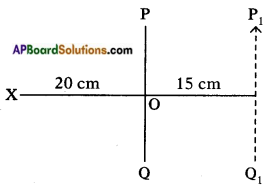AP State Syllabus AP Board 8th Class Physical Science Important Questions Chapter 10 Reflection of Light at Plane Surfaces

## AP State Syllabus 8th Class Physical Science Important Questions 10th Lesson Reflection of Light at Plane Surfaces

### 8th Class Physical Science 10th Lesson Reflection of Light at Plane Surfaces 1 Mark Important Questions and Answers

Question 1.
What is reflection?
The light rays falling on a surface are returned into the original medium. This phenomenon is called reflection.Question 2.
How Is image appeared with a big hole of pinhole camera?
We get blurred image on the screen due to big hole of the camera.

Question 3.
Which path light tends to prefer?
Light selects the path which takes least time to travel.

Question 4.
What is meant by lateral inversion?
The right appears as left in the image is called lateral inversion.

Question 5.
If an object placed in front of a plane mirror has size 6 cm, then what is size of the image?
Size of the object = Size of image.
∴ Size of the image = 6 cm.

Question 6.
What is a real image? What is a virtual image?
Real image: The image formed due to convergence of light rays. The real image can be caught on the screen.
Virtual image: The image that we get by extending the rays backwards is called a virtual image. A virtual image cannot be caught on the screen.

Question 7.
What is a reflecting surface?
The surface used for reflection is called reflecting surface.Question 8.
What is principal axis?
The horizontal line which passes through the centre of curvature is called principal axis.

Question 9.
What is meant by converging of light rays?
If light rays after reflection meet at a point, then we say the light rays are converging.

Question 10.
Why does our image appear thin or bulged?
Due to converging or diverging of light rays from the mirror.

Question 11.
Why is angle of incidence equal to angle of reflection when a light ray reflects from a surface?
Because light selects the path that takes least time to cover a distance.

Question 12.
Are angle of reflection and angle of incidence also equal for curved surface?
Yes, it is equal for curved surfaces like spherical mirrors.

Question 13.
Why is there right-left inversion (lateral inversion) when we look into mirror?
(OR)
Explain the lateral (righ-left) inversion of the image in plane mirrors through an example.

1. The light rays which come from object get reflected from the plane mirror and reach our eye.
2. Our brain feels that the ray is coming from the inside of mirror.
3. So there is right-left inversion.Question 14.
What are the minimum conditions to get shadows?
The minimum conditions required are a source of light to produce light, an opaque object to obstruct the light and a screen to collect the shadow.

Question 15.
What happens if we increase the size of the hole of the pinhole camera?
If the size of the pinhole camera is increased, the image seems to be blurred.

Question 16.
Name some apparatus which can work on the principle of reflection of light.
Plane mirror, spherical mirrors, periscope, kaleidoscope.

Question 17.
What is your opinion on elevating buddings with mirrors?
The mirrors used in elevating buildings are reinforced, tough and laminated glasses. These mirrors provide safety and make the buildings attractive.

Question 18.
What happens when light falls on an opaque object?
Some part of light is reflected back and remaining part is absorbed.

Question 19.
What happens when light is reflected from transparent object?
Some part of light is reflected and remaining part is partly transmitted or partly absorbed.

Question 20.
If angle of incidence of light ray on a plane mirror is 40°, then what is the angle between incident ray and reflected ray?
The angle between incident ray and reflected ray is 80°.Question 21.
From the figure, find angle of reflection.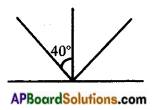The angle of reflection is 40°.

Question 22.
If distance of plane mirror and object is 10 cm, then what is the distance between object and its image ?
Object distance = image distance
∴ Distance between object and image = 10 + 10 = 20 cms.

Question 23.
If the angle between the mirror and incident ray is 40°, then find the angle of reflection.
Given that angle between incident ray and mirror = 40°.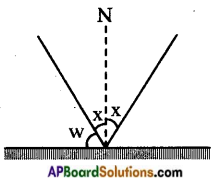Suppose angle of incidence = x.
∴ 40 + x = 90
x = 90 – 40 = 50°.
But we know angle of incidence = angle of reflection
Angle of reflection = 50°.

Question 24.
State Fermat’s principle.
Light chooses the path which takes the least time to travel.Question 25.
Can a virtual image be photographed by a camera?
Yes, virtual image can be photographed by a camera.

### 8th Class Physical Science 10th Lesson Reflection of Light at Plane Surfaces 2 Marks Important Questions and Answers

Question 1.
What are the characteristics of image formed by a plane mirror?
Characteristics of image formed by a plane mirror.

1. It is a virtual image.
2. The image is straight.
3. The image is laterally inverted.
4. Size of the image is equal to size of the object.
5. Image distance is equal to object distance.

Question 2.
Can a plane mirror ever form a real image?

1. Real image can only be formed when the reflected rays converge.
2. For plane mirrors it is not possible.
3. However if the reflected rays are converged it can form a reed image.
4. Consider a source of light at infinity (say sun) and plane mirror is very small in size, its reflected image will show a circular bright spot (image of sun) on the screen.Question 3.
Frame some questions on the importance of ‘perpendicular’ in the reflection of light.

1. Why do we require perpendicular (normal) to a plane mirror?
2. If light falls normal to the surface of a plane mirror, what happens?
3. What is the importance of normal?
4. In this figure what is angle of reflection?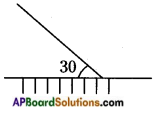Question 4.
How is an image formed by a plane mirror?

1. ‘O’ is a point object. Some rays from O reach the mirror and get reflected.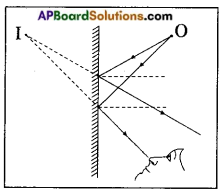2. When we look into the mirror, the reflected rays seem to be coming from the point I. So point I is the image of point object ‘O’.

Question 5.
Draw the ray diagram of lateral inversion of a letter.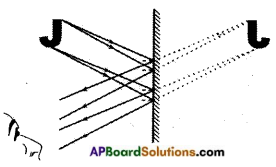Question 6.
Draw the ray diagram showing point object (O). (OR)
Draw the ray diagram showing an object (OO’) by a plane mirror.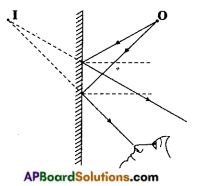Question 7.
Observe the given figure. Write the values of angle of incidence and angle of reflection. Complete the figure using these values.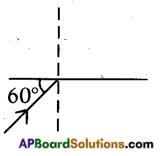The angle of incidence (i) = 90 – 60 = 30°
The angle of reflection (r) = 30°
(∵ angle of incidence = angle of reflection)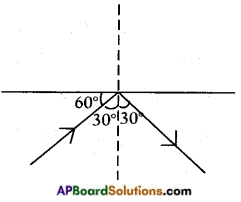Question 8.
Draw the diagram that explains the formation of an Image by a plane mirror.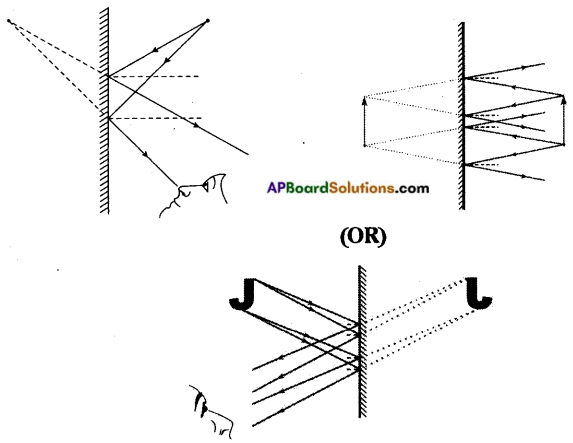### 8th Class Physical Science 10th Lesson Reflection of Light at Plane Surfaces 4 Marks Important Questions and Answers

Question 1.
Write briefly about formation of image by a plane mirror. Write characteristics of image. (OR)
A teacher asked a student which mirror is used by him to see his image at home. Then name that mirror and also give formation of image due to that mirror and characteristic of image formed by that mirror.
Image formation for a point sized object:

1. ‘O’ is a point object. Some rays from ‘O’ reach mirror and get reflected.
2. When we look into the mirror, the reflected rays seem to be coming from the point I.
3. So T is the image of point ‘O’.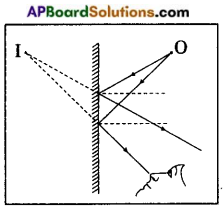Image formation of an object:
4. Now place an erect object in front of plane mirror.
5. Draw some incident rays from the object to the mirror and reflected rays from the mirror using laws of reflection.
6. The rays coming from the point ‘O’ and reflected from the mirror seem to be coming from point ‘I’.
7. So I is the image of ‘O’.
8. The rays coming from the point O1 get reflected from the mirror and seems to be coming from point I1. So I1 is the image of O1.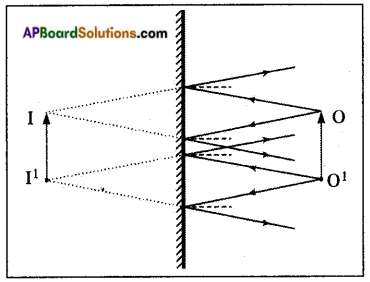9. The rays coming from the middle part of the O and O1 will form their images between I and I1.
10. Thus I – I1 is the image of the object O – O1.

Characteristics of image:

1. The image has same size as object.
2. The image distance and object distance are same.
3. The image undergoes right-left inversion (lateral inversion).
4. The image is virtual and erect.Question 2.
Why does the size of the image decrease when you move the object towards your eye?

1. The given figure shows how our eye judges the distance of an object.
2. The object which is at point ‘O’ looks smaller to the observer 2 than to the observer 1 because the light rays coming from the object 1 makes a smaller angle at the eye of the observer 2 compared to observer 1.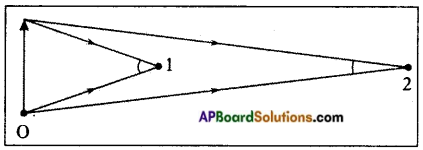3. The angle plays the role in sensing the size of the object.
4. Similarly, when we move the object from the mirror to our eye, the image in the mirror seems to move back in the mirror.
5. Then the distance from the image to our eye increases.
6. The angle formed by image at our eye is smaller than that of angle formed by the object.
7. That is why the image looks smaller than the object.

Question 3.
Why does a plane mirror act as better reflecting surface when compared with other reflecting surfaces? Why?

1. When light falls on any surface some part of light is reflected by the surface, some other part is transmitted by the surface and remaining part is absorbed by the surface.
2. However, a reflecting surface like mirror reflects most of the light (nearly 90%) fallen on it.
3. A plane mirror is made by polishing or silvering one side of plane glass.
4. The side which is silvered called silvered surface while the other side is called reflecting surface.
5. The light always reflects from reflecting surface.
6. To protect the silver polish or silvered surface often it is painted with red or black colours.
7. This type of arrangement actually increases the reflection up to 90%. So it acts as better reflecting surface.Question 4.
The figure shows a plane mirror PQ at distance 20 cm from an object. The mirror is moved 15 cm away from object O to next position. What is the shift in the image of O?